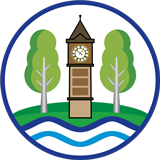School Performance

2018 KS2 SAT Results

Expected standard in Reading = 72%

Greater Depth in Reading = 15%

Expected standard in Maths = 65%

Greater Depth in Maths = 20%

Expected standard in Writing = 72%

Greater Depth in Writing = 0%

The number of children achieving the expected standard or above in reading, writing and maths (combined) = 58%

The number of children achieving a high level of attainment in reading, writing and maths (combined) = 0%

The average progress that children make in reading = -2.7

The average progress that children make in writing = -2.6

The average progress that children make in maths = -0.2

The average 'scaled score' in reading = 102.0

The average 'scaled score' in maths = 103.4

****************************************************************************

2017 KS2 SAT Results

The number of children achieving the expected standard or above in reading, writing and maths (combined) = 40%

The number of children achieving a high level of attainment in reading, writing and maths (combined) = 3%

The average progress that children make in reading = -3.7

The average progress that children make in writing = 1.3

The average progress that children make in maths = -2.9

The average 'scaled score' in reading = 99

The average 'scaled score' in maths = 99